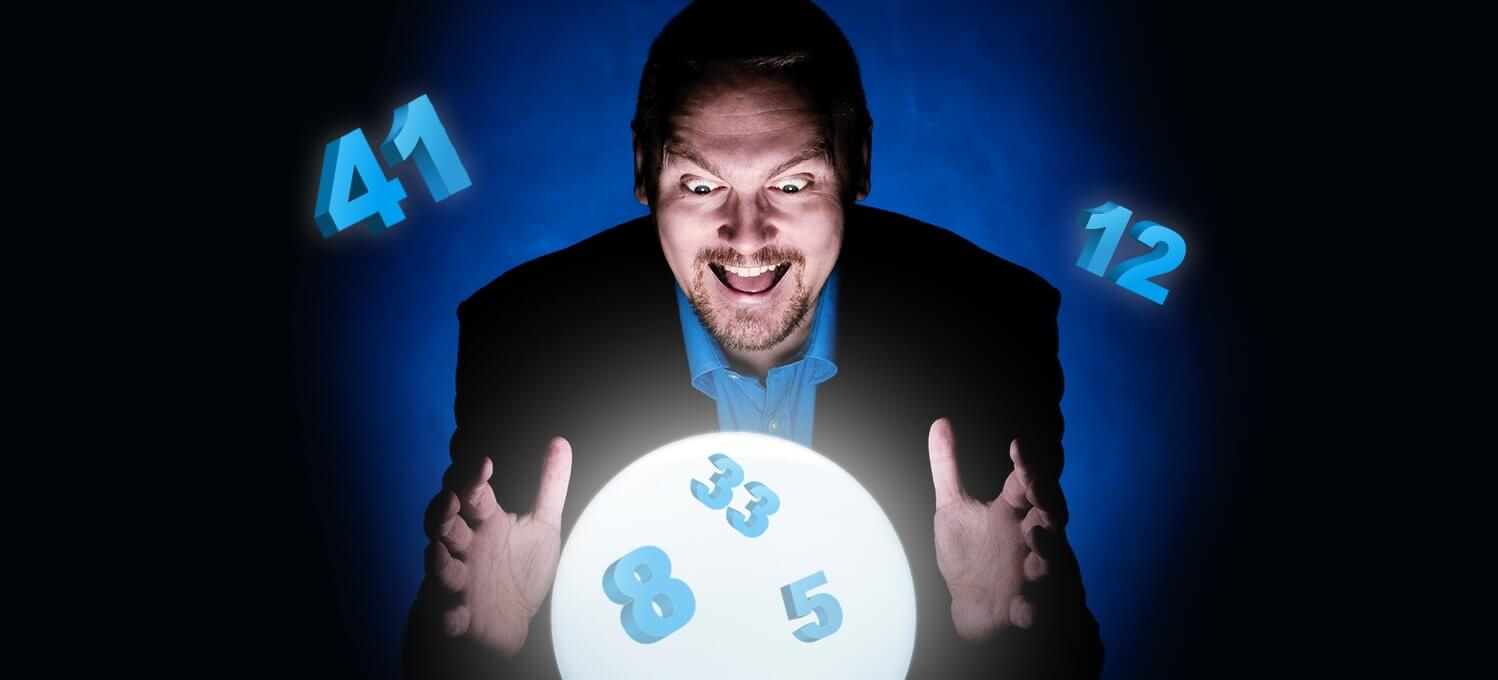# Can Lottery Numbers Be Predicted?

• Updated
• By Katie ThompsonThe outcomes of many gambling-led games can, to a degree, be predicted. Take, for example, world-class blackjack players counting cards, or even a skilled mathematician trying to predict the odds on a roulette wheel.

But while the latter may seem almost impossible, there are other forms of gaming which seem absolutely impossible to predict. Did you know, for example, that you are four times more likely to be struck by lightning than you are to win the lottery?

Ridiculous odds aside, there are a few optimistic folks who do in fact believe that there may be more to lottery outcomes than we previously thought.

So, when it comes to thinking about whether or not the lottery can be predicted, the answer may not be so simple: in fact, it may come down to a question of mathematics.

## Predicting the lottery numbers

First things first – it’s important to remember that you'll never be able to pick the exact numbers, in the correct sequence. However, the laws of mathematics dictate that you should, at least in theory, be able to predict which numbers appear more often than others.

### The principles of probability

Generally speaking, it is thought that any combination of numbers is as likely to come up as any other. However, did you know that the consecutive numbers 1,2,3,4,5 and 6 have never been drawn?

This is no coincidence – it is controlled by probability, which is affected by both independent events and dependent events. For example, if you had a bag with 90 red snooker balls and 10 snooker balls and you took one out and replaced it, you would more likely pick a red ball every time if you returned it every time.

Put simply, probability is an equation, equalling the number of favourable items to one outcome (let’s say the red balls) divided by the total number of outcomes. So in this case, the probability for drawing a red ball would be nine out of 10 draws, and yellow, one out of 10.

### Applying this to the lottery

The next part involves some very tricky maths – a binomial coefficient – which we'll simplify below. Let us say there is a lottery with 45 numbers and six combinations – this would give us 8,145,060 different outcomes.

So, based on our formula, if we were assessing the odds of three odds and three evens, the number of combinations would be 2,727,340.

Therefore, the probability of achieving three odds and three even numbers is P (3 odd and even) 0.33484590659. Based on the abovementioned formulae, the other six patterns e.g. four odd and two even etc. return smaller values, so the biggest probability is a combination of three odds and three evens.

Of course, this calculation will not help you to predict the right six numbers, but it does demonstrate that mathematics tells us we are more likely to win with three even numbers and three odd numbers. Try it yourself!